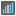• # decimal point trimming

## Question related to missionMedian

This seems like a bug to me, but it could be me. It seems like my decimal points are being lopped off when I attempt to return a float value (as seen in the return float(12.5) below.

I would like to give some feedback about ...

HTTP_USER_AGENT:

```Mozilla/5.0 (Windows NT 6.1; WOW64; rv:31.0) Gecko/20100101 Firefox/31.0
```

My Code:

```def checkio(data):

srt = sorted(data)
l = len(data)

print (srt)

if l % 2 == 1:
return srt[l // 2]
else:
print (srt[l // 2] , "+", srt[l // 2 - 1])
x = srt[(l // 2)]
y = srt[(l // 2 - 1)]

if (x + y)//2.0 == 12.5:
**return float(12.5)**
return (x + y)//2.0

#replace this for solution
return 'ERROR'

#These "asserts" using only for self-checking and not necessary for auto-testing
if __name__ == '__main__':
assert checkio([1, 2, 3, 4, 5]) == 3, "Sorted list"
assert checkio([3, 1, 2, 5, 3]) == 3, "Not sorted list"
assert checkio([1, 300, 2, 200, 1]) == 2, "It's not an average"
assert checkio([3, 6, 20, 99, 10, 15]) == 12.5, "Even length"
print("Start the long test")
assert checkio(list(range(1000000))) == 499999.5, "Long."
print("The local tests are done.")
```